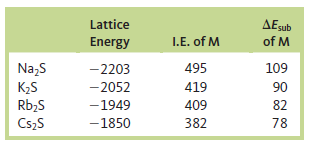# Problem: Use the following data (in kJ/mol) to estimate ΔE for the reaction S  -(g) + e- → S2-(g). Include an estimate of uncertainty. ΔEsub is the energy of sublimation.2 Na(s) + S(s) → Na 2S(s)      ΔE = -365 kJ/mol  2 K(s) + S(s) → K 2S(s)         ΔE = -381 kJ/mol2 Rb(s) + S(s) → Rb 2S(s)      ΔE = -361 kJ/mol2 Cs (s) + S(s) → Cs 2S(s)     ΔE = -360 kJ/mol                 S(s) → S(g)           ΔE = 277 kJ/mol          S(g) + e - → S-(g)         ΔE = -200 kJ/molAssume that all values are known to +1 kJ/mol.

###### FREE Expert Solution
100% (243 ratings)
###### Problem Details

Use the following data (in kJ/mol) to estimate ΔE for the reaction S  -(g) + e- → S2-(g). Include an estimate of uncertainty. ΔEsub is the energy of sublimation.2 Na(s) + S(s) → Na 2S(s)      ΔE = -365 kJ/mol
2 K(s) + S(s) → K 2S(s)         ΔE = -381 kJ/mol
2 Rb(s) + S(s) → Rb 2S(s)      ΔE = -361 kJ/mol
2 Cs (s) + S(s) → Cs 2S(s)     ΔE = -360 kJ/mol
S(s) → S(g)           ΔE = 277 kJ/mol
S(g) + e → S-(g)         ΔE = -200 kJ/mol

Assume that all values are known to +1 kJ/mol.

Frequently Asked Questions

What scientific concept do you need to know in order to solve this problem?

Our tutors have indicated that to solve this problem you will need to apply the Born Haber Cycle concept. You can view video lessons to learn Born Haber Cycle. Or if you need more Born Haber Cycle practice, you can also practice Born Haber Cycle practice problems.

What textbook is this problem found in?

Our data indicates that this problem or a close variation was asked in Chemistry: An Atoms First Approach - Zumdahl Atoms 1st 2nd Edition. You can also practice Chemistry: An Atoms First Approach - Zumdahl Atoms 1st 2nd Edition practice problems.